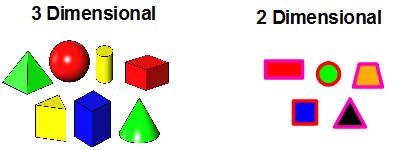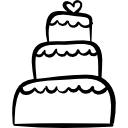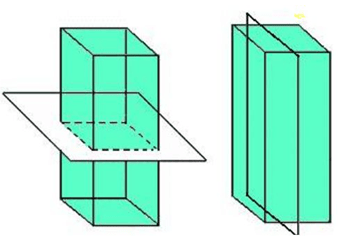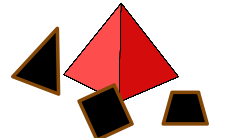# Cross Sections

In this lesson, we will learn how to identify and describe two-dimensional figures known as cross-sections that result from slicing three-dimensional figures. In other words, you can slice a three-dimensional figure (rectangular prism, pyramid, cone, cylinder, and sphere) to show a two-dimensional view (rectangle, square, triangle, circle, trapezoid…). The two-dimensional view is called a cross-section.Let us begin with a shape that many of you have seen before – a cake! Think about it. Did you know that many cakes are shaped like cylinders? Look at the cake below. It appears to be round on top but what happens when you cut yourself a slice?

Can you see the rectangle on the inside? When you slice a cake it is the same as a cross-section. The cross-section is simply a view of the inside of a three-dimensional figure after it is sliced. In mathematics, we try to visualize planes cutting across the three-dimensional figures. There are several ways to cut a three-dimensional figure that rest on a base:

## Example 1

Let us look at a rectangular prism.

As it rests on its rectangular base, we cut it with a plane Parallel to that base. The cross-section is shaped like a rectangle. It has the same shape and size as the base.

Now, we will slice it with a plane perpendicular to the base. Remember, perpendicular lines intersect the base at ninety degrees. Again, the cross-section is shaped like a rectangle. However, this time it has the same shape and size as another face of the prism.

Think about slicing the prism with a plane tilted away from its base. This time, the cross-section will be shaped like a parallelogram.## Example 2

Another example of a cross-section would be one that is formed when we slice a cone through its vertex. Of course, the cone sits on its circular base. If you cut it perpendicular to its base and through its vertex, the cross-section would be shaped like an isosceles triangle. The base of the triangle will be the base of the cone.

The cone would create a circle shape if the plane sliced it parallel to its base## Example 3

Next, let’s picture a three-dimensional figure that has one base shaped like a polygon (a plane figure with at least three straight sides and angles) and the other faces shaped like triangles that share a common vertex. Can you guess what this is?A pyramid is named for the shape of its base. Let us look at a square pyramid (has a square base). Imagine a vertical plane cutting through the pyramid perpendicular to that base. The cross-section would be shaped like a triangle. If you sliced the pyramid parallel to the base, the cross-section would be shaped like a square (base). Now, cut the pyramid perpendicular to the base, but NOT at the vertex. This will give you a trapezoid!

Remember! When you slice any shape parallel to its base, you will ALWAYS get a figure that is the shape of the base.

Watch the videos to see some interactive examples of different types of cross-sections that can be created from cubes and pyramids.

## Match the Slice with the Shape Practice

#### Match the description to the shape.

1. Slice a rectangular prism parallel to its base.
2. Slice a cube perpendicular to its base.
3. Slice a hexagonal pyramid through its vertex perpendicular to its base.
4. Slice a square pyramid perpendicular to the base and not through the vertex.
5. Slice a cylinder parallel to its base.
6. Slice a cube slightly tilted neither horizontal nor vertical to the faces.

(source)